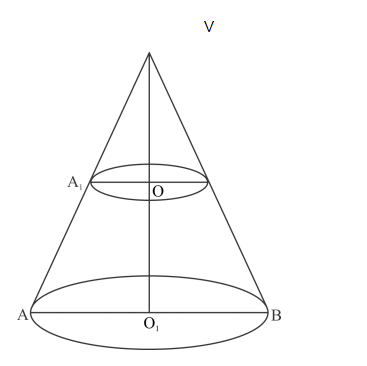# The height of a cone is 20 cm.Question:

The height of a cone is 20 cm. A small cone is cut off from the top by a plane parallel to the base. If its volume be 1/125 of the volume of the original cone, determine at what height above the base the section is made.

Solution:

We have the following situation as shown in the figureLet VAB be a cone of height h1 VO1 =20cm. Then from the symmetric triangles VO 1A and VOA1, we have

$\frac{\mathrm{VO}_{1}}{\mathrm{VO}}=\frac{\mathrm{O}_{1} \mathrm{~A}}{\mathrm{OA}_{1}}$

$\Rightarrow \frac{20}{V O}=\frac{O_{1} A}{O A_{1}}$

It is given that, volume of the cone $\mathrm{VA}_{1} \mathrm{O}$ is $\frac{1}{125}$ times the volume of the cone $\mathrm{VAB}$. Hence, we have

$\frac{1}{3} \pi \mathrm{OA}_{1}{ }^{2} \times \mathrm{VO}=\frac{1}{125} \times \frac{1}{3} \pi \mathrm{O}_{1} \mathrm{~A}^{2} \times 20$

$\Rightarrow\left(\frac{\mathrm{OA}_{1}}{\mathrm{O}_{1} \mathrm{~A}}\right)^{2} \times \mathrm{VO}=\frac{4}{25}$

$\Rightarrow\left(\frac{\mathrm{VO}}{20}\right)^{2} \times \mathrm{VO}=\frac{4}{25}$

$\Rightarrow \quad \mathrm{VO}^{3}=\frac{400 \times 4}{25}$

$\Rightarrow \quad \mathrm{VO}^{3}=16 \times 4$

$\Rightarrow \quad \mathrm{VO}=4$

Hence, the height at which the section is made is 20 − 4 = 16 cm.